##### Finite Math For Dummies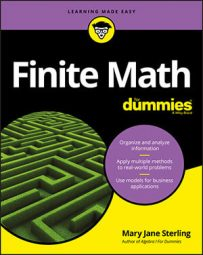If your finite math instructor asks you to analyze a compound statement, you can try using a truth table to do this. Not every topic in a discussion can be turned into a compound statement and analyzed for its truth that way, but using logic and truth values is a good technique to use when possible.

Consider the compound statement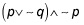When constructing a truth table, you start with the basic p and q columns. Then you add a ~ q column followed by a column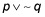Before you can perform the conjunction, ^, you need a ~ p column. Here’s a step-by-step procedure.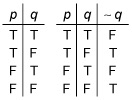column, perform the disjunction on the first and third columns. Remember, with disjunctions, the statement is false only when both component statements are false.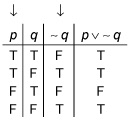3. Add the ~ p column.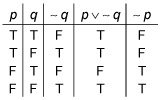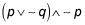column, which shows the conjunction of the fourth and fifth columns.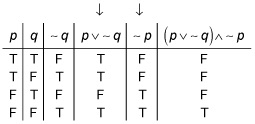The conjunction is true only when the two component statements are true. This complex statement is only true when both original statements are false.